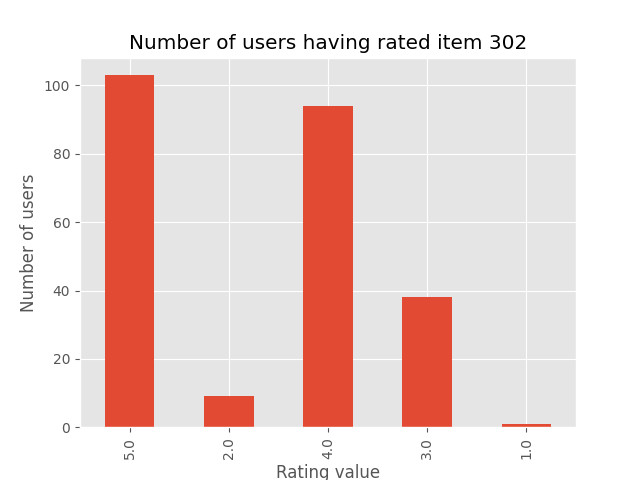# Analysis of the KNNBasic algorithm¶

In this notebook, we will run a basic neighborhood algorithm on the movielens dataset, dump the results, and use pandas to make some data analysis.

In :
from __future__ import (absolute_import, division, print_function,
unicode_literals)
import pickle
import os

import pandas as pd

from surprise import KNNBasic
from surprise import Dataset
from surprise.model_selection import PredefinedKFold
from surprise import dump
from surprise.accuracy import rmse

In :
# We will train and test on the u1.base and u1.test files of the movielens-100k dataset.
# You can do it manually, or by running:

# Now, let's load the dataset
train_file = os.path.expanduser('~') + '/.surprise_data/ml-100k/ml-100k/u1.base'
test_file = os.path.expanduser('~') + '/.surprise_data/ml-100k/ml-100k/u1.test'

pkf = PredefinedKFold()

# We'll use a basic nearest neighbor approach, where similarities are computed
# between users.
algo = KNNBasic()

for trainset, testset in pkf.split(data):
algo.fit(trainset)
predictions = algo.test(testset)
rmse(predictions)

dump.dump('./dump_file', predictions, algo)

Computing the msd similarity matrix...
Done computing similarity matrix.
RMSE: 0.9889

In :
# The dump has been saved and we can now use it whenever we want.
# Let's load it and see what we can do

In :
trainset = algo.trainset
print('algo: {0}, k = {1}, min_k = {2}'.format(algo.__class__.__name__, algo.k, algo.min_k))

algo: KNNBasic, k = 40, min_k = 1

In :
# Let's build a pandas dataframe with all the predictions

def get_Iu(uid):
"""Return the number of items rated by given user

Args:
uid: The raw id of the user.
Returns:
The number of items rated by the user.
"""

try:
return len(trainset.ur[trainset.to_inner_uid(uid)])
except ValueError:  # user was not part of the trainset
return 0

def get_Ui(iid):
"""Return the number of users that have rated given item

Args:
iid: The raw id of the item.
Returns:
The number of users that have rated the item.
"""

try:
return len(trainset.ir[trainset.to_inner_iid(iid)])
except ValueError:  # item was not part of the trainset
return 0

df = pd.DataFrame(predictions, columns=['uid', 'iid', 'rui', 'est', 'details'])
df['Iu'] = df.uid.apply(get_Iu)
df['Ui'] = df.iid.apply(get_Ui)
df['err'] = abs(df.est - df.rui)

In :
df.head()

Out:
uid iid rui est details Iu Ui err
0 1 6 5.0 3.468613 {'actual_k': 20, 'was_impossible': False} 135 20 1.531387
1 1 10 3.0 3.866290 {'actual_k': 40, 'was_impossible': False} 135 73 0.866290
2 1 12 5.0 4.538194 {'actual_k': 40, 'was_impossible': False} 135 211 0.461806
3 1 14 5.0 4.235741 {'actual_k': 40, 'was_impossible': False} 135 140 0.764259
4 1 17 3.0 3.228002 {'actual_k': 40, 'was_impossible': False} 135 72 0.228002
In :
best_predictions = df.sort_values(by='err')[:10]
worst_predictions = df.sort_values(by='err')[-10:]

In :
# Let's take a look at the best predictions of the algorithm
best_predictions

Out:
uid iid rui est details Iu Ui err
272 5 439 1.0 1.0 {'actual_k': 3, 'was_impossible': False} 91 3 0.0
886 13 314 1.0 1.0 {'actual_k': 2, 'was_impossible': False} 373 2 0.0
156 2 314 1.0 1.0 {'actual_k': 2, 'was_impossible': False} 40 2 0.0
926 13 437 1.0 1.0 {'actual_k': 3, 'was_impossible': False} 373 3 0.0
9276 206 314 1.0 1.0 {'actual_k': 1, 'was_impossible': False} 33 2 0.0
19118 405 437 1.0 1.0 {'actual_k': 3, 'was_impossible': False} 582 3 0.0
8032 181 1334 1.0 1.0 {'actual_k': 1, 'was_impossible': False} 218 1 0.0
8041 181 1354 1.0 1.0 {'actual_k': 1, 'was_impossible': False} 218 1 0.0
9202 201 1424 3.0 3.0 {'actual_k': 1, 'was_impossible': False} 215 1 0.0
3018 60 1123 4.0 4.0 {'actual_k': 1, 'was_impossible': False} 119 1 0.0

It's interesting to note that these perfect predictions are actually lucky shots: $|U_i|$ is always very small, meaning that very few users have rated the target item. This implies that the set of neighbors is very small (see the actual_k field)... And, it just happens that all the ratings from the neighbors are the same (and mostly, are equal to that of the target user).

This may be a bit surprising but these lucky shots are actually very important to the accuracy of the algorithm... Try running the same algorithm with a value of min_k equal to $10$. This means that if there are less than $10$ neighbors, the prediction is set to the mean of all ratings. You'll see your accuracy decrease!

In :
# Now, let's look at the prediction with the biggest error
worst_predictions

Out:
uid iid rui est details Iu Ui err
9406 208 302 1.0 4.308447 {'actual_k': 40, 'was_impossible': False} 11 245 3.308447
19089 405 169 1.0 4.364728 {'actual_k': 40, 'was_impossible': False} 582 97 3.364728
19785 436 132 1.0 4.365369 {'actual_k': 40, 'was_impossible': False} 126 200 3.365369
157 2 315 1.0 4.381308 {'actual_k': 40, 'was_impossible': False} 40 136 3.381308
8503 193 56 1.0 4.386478 {'actual_k': 40, 'was_impossible': False} 61 312 3.386478
5531 113 976 5.0 1.610771 {'actual_k': 7, 'was_impossible': False} 31 7 3.389229
7917 181 408 1.0 4.421499 {'actual_k': 40, 'was_impossible': False} 218 93 3.421499
7390 167 169 1.0 4.664991 {'actual_k': 40, 'was_impossible': False} 38 97 3.664991
7412 167 1306 5.0 1.000000 {'actual_k': 1, 'was_impossible': False} 38 1 4.000000
5553 114 1104 5.0 1.000000 {'actual_k': 1, 'was_impossible': False} 27 1 4.000000

Let's focus first on the last two predictions. Well, we can't do much about them. We should have predicted $5$, but the only available neighbor had a rating of $1$, so we were screwed. The only way to avoid this kind of errors would be to increase the min_k parameter, but it would actually worsen the accuracy (see note above).

How about the other ones? It seems that for each prediction, the users are some kind of outsiders: they rated their item with a rating of $1$ when the most of the ratings for the item where high (or inversely, rated a bad item with a rating of $5$). See the plot below as an illustration for the first rating.

These are situations where baseline estimates would be quite helpful, in order to deal with highly biased users (and items).

In :
from collections import Counter

import matplotlib.pyplot as plt
import matplotlib
%matplotlib notebook
matplotlib.style.use('ggplot')

counter = Counter([r for (_, r) in trainset.ir[trainset.to_inner_iid('302')]])
pd.DataFrame.from_dict(counter, orient='index').plot(kind='bar', legend=False)
plt.xlabel('Rating value')
plt.ylabel('Number of users')
plt.title('Number of users having rated item 302')Out:
Text(0.5, 1.0, 'Number of users having rated item 302')
In [ ]: